# 17-9 Answer true or false. (a) The one aldehyde and the one ketone with a molecular formula of C 3 H 6 O are constitutional isomers. (b) Aldehydes and ketones both contain a carbonyl group. (c) The VSEPR model predicts bond angles of 1200 about the carbonyl carbon of aldehydes and ketones. (d) The carbonyl carbon of a ketone is a stereocenter.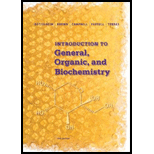### Introduction to General, Organic a...

11th Edition
Frederick A. Bettelheim + 4 others
Publisher: Cengage Learning
ISBN: 9781285869759### Introduction to General, Organic a...

11th Edition
Frederick A. Bettelheim + 4 others
Publisher: Cengage Learning
ISBN: 9781285869759

#### Solutions

Chapter 17, Problem 17.9P
Textbook Problem

## 17-9 Answer true or false.(a) The one aldehyde and the one ketone with a molecular formula of C3H6O are constitutional isomers.(b) Aldehydes and ketones both contain a carbonyl group.(c) The VSEPR model predicts bond angles of 1200 about the carbonyl carbon of aldehydes and ketones.(d) The carbonyl carbon of a ketone is a stereocenter.

Expert Solution

(a)

Interpretation Introduction

Interpretation:

Answer true or false for the following statement.

The one aldehyde and one ketone with a molecular formula of C3H6O are constitutional isomers.

Concept Introduction:

Constitutional isomers have the same molecular formula but different structural formulas or connectivity with atoms is different. In aldehydes, the carbonyl group is bonded to a hydrogen atom and ketones carbonyl group is bonded to two carbon atoms.

The one aldehyde and one ketone with a molecular formula of C3H6O are constitutional isomers is the true statement.

### Explanation of Solution

The given molecular formula: C3H6O

For this molecular formula we can draw both aldehyde and ketone. They are as follows.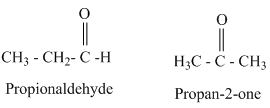From the above structures aldehydes and ketones having same molecular formula are constitutional isomers.

Therefore, the given statement is true.

Expert Solution

(b)

Interpretation Introduction

Interpretation:

Answer true or false for the following statement.

Aldehydes and ketones both contain a carbonyl group.

Concept Introduction:

In aldehydes, the carbonyl group is bonded to a hydrogen atom and ketones carbonyl group is bonded to two carbon atoms.

Aldehydes and ketones both contain a carbonyl group is true statement.

### Explanation of Solution

In aldehydes, the carbonyl group is bonded to a hydrogen atom and in ketone carbonyl group is bonded to two carbon atoms. Hence, both an aldehyde and a ketone have a carbonyl group.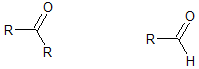Where, R is an alkyl group.

Therefore, the given statement is true.

Expert Solution

(c)

Interpretation Introduction

Interpretation:

Answer true or false for the following statement.

The VSEPR model predicts bond angles of 1200 about the carbonyl carbon of aldehydes and ketones.

Concept Introduction:

In aldehydes, the carbonyl group is bonded to a hydrogen atom and ketones carbonyl group is bonded to two carbon atoms. VSEPR model predicts the geometry of the molecule with the help of number of pair of electrons present around the central atoms and bond angle of the molecule.

The VSEPR model predicts bond angles of 1200 about the carbonyl carbon if aldehydes and ketones is the true statement.

### Explanation of Solution

In aldehydes, the carbonyl group is bonded to a hydrogen atom and ketones carbonyl group is bonded to two carbon atoms. The carbonyl group of aldehyde and ketone are represented are as follows.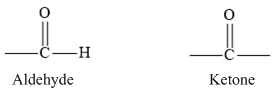According to VSEPR theory, three pair of electron groups forms a Trigonal planar geometry with bond angle of 1200.

Therefore, the given statement is true.

Expert Solution

(d)

Interpretation Introduction

Interpretation:

Answer true or false for the following statement.

The carbonyl carbon of ketone is a stereocentre.

Concept Introduction:

A stereocentre is a tetrahedral carbon atom in which four different groups are attached to it. When an atom generally carbon is linked with four different groups, then that centre is known as chiral centre.

The carbonyl carbon of ketone is a stereocenter is the false statement.

### Explanation of Solution

In aldehydes, the carbonyl group is bonded to a hydrogen atom and in ketone carbonyl group is bonded to two carbon atoms. The carbonyl group of aldehyde and ketone are represented are as follows.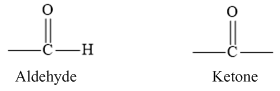In ketones, the carbonyl carbon is boded to carbonyl oxygen by a double bond group and bonded to two other carbon atoms.

Therefore, the given statement is false.

### Want to see more full solutions like this?

Subscribe now to access step-by-step solutions to millions of textbook problems written by subject matter experts!

### Want to see more full solutions like this?

Subscribe now to access step-by-step solutions to millions of textbook problems written by subject matter experts!